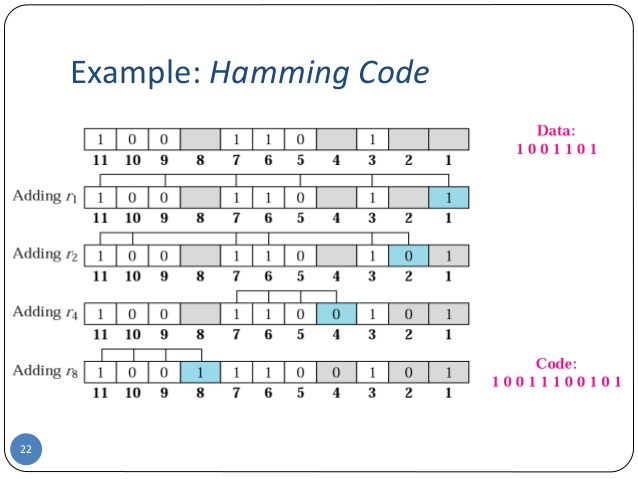# Hamming Code in C and C++

Here you will get program for hamming code in C and C++.

Hamming code is a popular error detection and error correction method in data communication. Hamming code can only detect 2 bit error and correct a single bit error which means it is unable to correct burst errors if may occur while transmission of data.

Also Read: Checksum Program in C and C++

Hamming code uses redundant bits (extra bits) which are calculated according to the below formula:-

2r ≥ m+r+1

Where r is the number of redundant bits required and m is the number of data bits.

R is calculated by putting r = 1, 2, 3 … until the above equation becomes true.

R1 bit is appended at position 20

R2 bit is appended at position 21

R3 bit is appended at position 22 and so on.

These redundant bits are then added to the original data for the calculation of error at receiver’s end.

At receiver’s end with the help of even parity (generally) the erroneous bit position is identified and since data is in binary we take complement of the erroneous bit position to correct received data.

Respective index parity is calculated for r1, r2, r3, r4 and so on.Image Source

1. Easy to encode and decode data at both sender and receiver end.
2. Easy to implement.

1. Cannot correct burst errors.
2. Redundant bits are also sent with the data therefore it requires more bandwidth to send the data.

## Program for Hamming Code in C++

Output

Enter 4 bits of data one by one
1
0
1
0

Encoded data is
1010010

Enter received data bits one by one
1
0
1
0
0
1
0

No error while transmission of data

Comment below if you have any queries related to above hamming code program in C and C++.

### 14 thoughts on “Hamming Code in C and C++”

1.This is the correct code please refer this:—-

#include
#include
void main() {
int data;
int dataatrec,c,c1,c2,c3,i;
clrscr();
printf(“Enter 4 bits of data one by one\n”);
scanf(“%d”,&data);
scanf(“%d”,&data);
scanf(“%d”,&data);
scanf(“%d”,&data);

data=data^data^data;
data=data^data^data;
data=data^data^data;

printf(“\nEncoded data is\n”);
for(i=0;i<7;i++)
printf("%d",data[i]);

printf("\n\nEnter received data bits one by one\n");
for(i=0;i<7;i++)
scanf("%d",&dataatrec[i]);

c1=dataatrec^dataatrec^dataatrec^dataatrec;
c2=dataatrec^dataatrec^dataatrec^dataatrec;
c3=dataatrec^dataatrec^dataatrec^dataatrec;
c=c3*4+c2*2+c1 ;

if(c==0 && dataatrec==data && dataatrec==data && dataatrec==data ) {
printf("\nNo error while transmission of data\n");
}
else {
printf("\nError on position %d",c);

printf("\nData sent : ");
for(i=0;i<7;i++)
printf("%d",data[i]);

for(i=0;i<7;i++)
printf("%d",dataatrec[i]);

printf("\nCorrect message is\n");

if(dataatrec[7-c]==0)
dataatrec[7-c]=1;
else
dataatrec[7-c]=0;

for (i=0;i<7;i++) {
printf("%d",data[i]);
}
}
}

1.Any exceptions ? Why ur adding if(c==0 && dataatrec==data && dataatrec==data && dataatrec==data )

2.1.It’s working just fine

2.wonderful boss! keep it up….

3.i need Left factoring Elimination program urgently..please do asap!!

4.I need a program in C for FIR filter.Showing its impulse response and magnitude response

5.ABHISHEK SRIVASTAV

Zero errors

6.i am enter 1111 as sent data and it shows that the ecoded data is 1111111. Fine but when i give it 1001011 as received data it shows me that there is No error while transmission of data.

1.Bro that’s cause hamming code can handle only single bits of data

7.This is showing 12 errors.

8.I Have problem use hamming code encoder send 4 bits 0001 to encoder output 0000111 go to channel 7 bits 1000111 use Binary Symmetric Channel end to decoder output 4 bit some input 0001. and my problem is how to write program to create BSC use c++ please share source code.

Thanks

9.Input: A binary message
Output: The codeword length if the message is transmitted using hamming code and the position of the parity bits (string of 0’s and 1’s)
How to write a program in c/c++?

10.Thank you! Your code worked greatly and helped a lot in our assignment.